Statistical analysis is the basis of modern life. Statistical analysis has allowed us to create powerful medicines that cure disease. They have allowed us to create cars that are safe, products that meet our needs and corporations that offer services that people only dreamed about a century ago. Almost every organization today uses statistical analysis to ensure profitability. And yet most people feel that statistical analysis is intimidating. This article will show you how simple and practical statistics can be by illustrating a simple linear regression example. The Introductory statistics, Part1: Descriptive Statistics will teach you how to use descriptive statistical analysis techniques so that you can summarize and analyze your own data.

Simple linear regression is a technique that displays the relationship between variable “y” based on the values of variable “x”. In simple terms we use linear regression relationships all the time in our own lives. We know as the temperature drops people put on more jackets to keep warm or as the gas price increases more people drive less to save money. In fact, economists rely on these relationships to manage the economy by increasing bank rates to discourage lending for example.

This example will explain linear regression in terms of students and their grades. Take a look at the following spreadsheet example: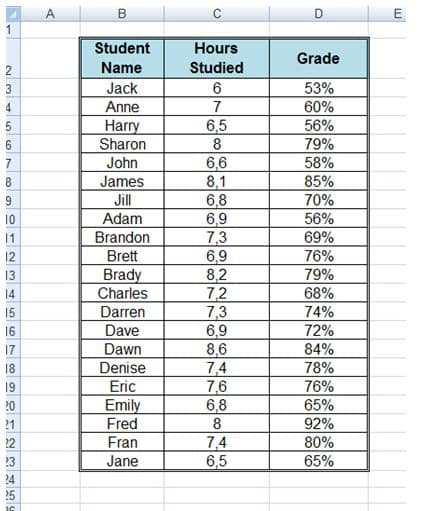This spreadsheet shows the number of hours a student studied and the grades achieved by the students. If we use a graph to represent the above table it would look like this: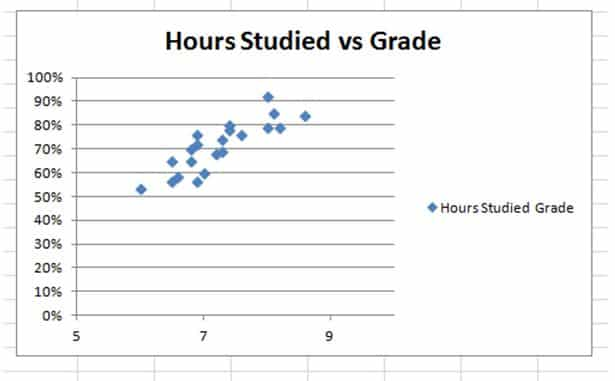The graph displays a definite upward trend indicating that the more hours a student studies, the more likely the student is to achieve a better result.

## How to Calculate Linear Regression

A regression in statistics is statistical analysis that assess the relationship between two variables. The calculation is used to determine the relationship between two variables. In our example, we need to determine the relationship between hours studied and grades achieved so that we can calculate what grade a student will achieve if they study for 9 hours for example.

What we are really looking for is a line that represents the average of the data above on the graph. We could add a line to the graph manually, but the results would not be as accurate as actually calculating the relationship.

The Equation for regression can be expressed as follows:

Regression Equation(y) = a + bx

Slope(b) = (NΣXY – (ΣX)(ΣY)) / (NΣX2 – (ΣX)2)

Intercept(a) = (ΣY – b(ΣX)) / N

Where:

x and y are the variables.

b = The slope of the regression line

a = The intercept point of the regression line and the y axis.

N = Number of values or elements

X = First Score

Y = Second Score

ΣXY = Sum of the product of first and Second Scores

ΣX = Sum of First Scores

ΣY = Sum of Second Scores

ΣX2 = Sum of square First Scores

The course in Probability and Statistics will take you through a step by step tutorial on how to calculate linear regression. The workshop will teach you probability, sampling, regression, and decision analysis and by the end of the workshop and you should be able to pass any introductory statistics course.

## Our Linear Regression Example using Excel

Excel offers a number of different functions that allow us to statically analyze data. To create a regression analysis of the above data, we need to select the “Data Analysis” option from the “Data” tab: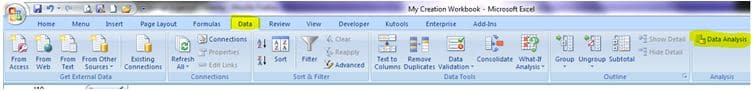Then select “Regression” from the Data Analysis options: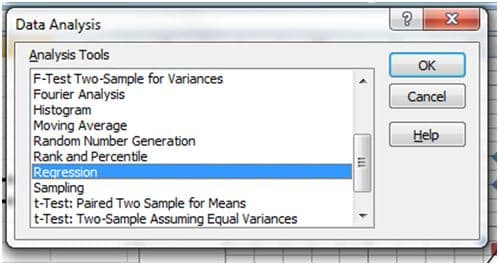Now all you need to do is indicate the x and Y values and indicated the output and chart type you want to draw: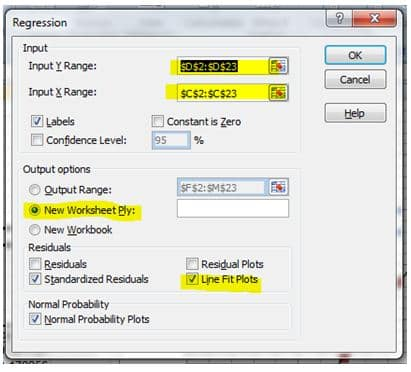Excel will perform the regression analysis for you and you will end up with a graph like this in the new worksheet to represent the linear regression:Now we can use the graph to make predictions about grades depending on how long a student studies for a test: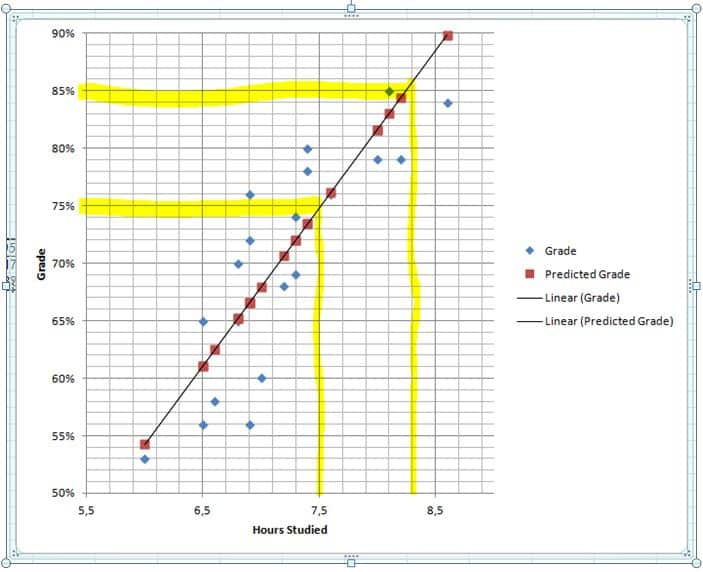Using the graph we can predict that a student who studies for about 7 and a half hours should get a grade of about 75% for this test and a student who studies for about 8.3 hours should get a grade of about 85%.

## Practical Statistics

We’ve used a simple example to show you how simple statistics can be, but statistics are becoming more and more important to ensure businesses make the right decisions to remain profitable. Statistics are used to determine which adverts are best, what products will succeed and how a company should spend its money. As companies realize the power of the internet, they are also realizing that user experience of their products can severely impact their profit margins. Statistics are therefore being used to ensure optimum user experience too. The Practical Statistics for The User Experience I course offers easy to understand concepts and lots examples that will help you generate statistical solutions to common questions in user research.

Page Last Updated: March 2014

### Top courses in Excel

Microsoft Excel - Excel from Beginner to Advanced
Kyle Pew, Office Newb
4.6 (400,246)
Bestseller
Microsoft Excel: Data Analysis with Excel Pivot Tables
Maven Analytics, Chris Dutton
4.6 (55,191)
Bestseller
Microsoft Excel: Data Visualization, Excel Charts & Graphs
Maven Analytics, Chris Dutton
4.6 (42,917)
Bestseller
Microsoft Excel Pro Tips: Go from Beginner to Advanced Excel
Maven Analytics, Chris Dutton
4.6 (4,965)
Bestseller
Microsoft Excel: Beginner to Advanced for 2023
4.6 (2,207)
Microsoft Excel - Excel from Beginner to Advanced 2023
Warrick Klimaytys
4.6 (8,041)
Bestseller
Microsoft Excel Certification Exam Prep: MO-201 Excel Expert
Maven Analytics, Enrique Ruiz
4.7 (1,888)
Bestseller